## General Mechanical

•Mahdi_171
Subscriber

Hi,

I have been trying to simulate the compression of a car jounce bumper (bump stop) for my graduation project. The problem is that I have to fully compress it (till solid height) and the hyperelastic model is not converging far before that.

•Erik Kostson
Ansys Employee
I would start with running this as 2D axisymmetric - it will run faster and be easier faster to change things to get convergence.nnErikn
•Mahdi_171
Subscriber
Dear Erik,nThank you for your quick response.nnI have been trying to work with a 2D axisymmetric model for the past days and I put all the body edges as targets and contacts with frictionless behaviour (I don't know if this is the right way to model self-contact or not). It did actually converge to a further point with mixed formulation, and reduced integration commands only.n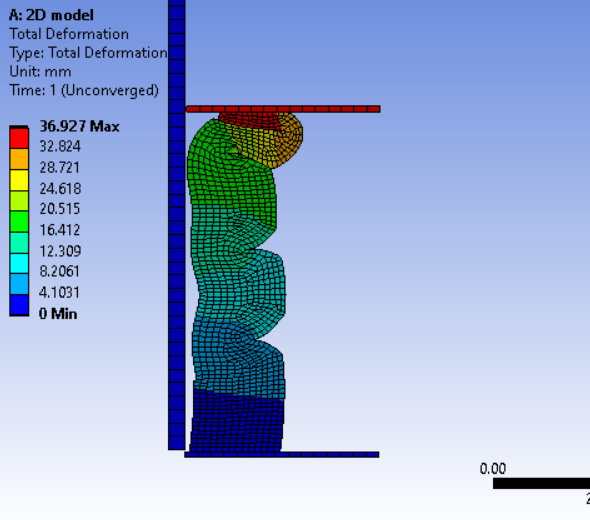nHowever, not till all the layers are in contact as I wish. I saw from the newton-raphson residuals force plots that the issue is at some to the folded edges in contact.n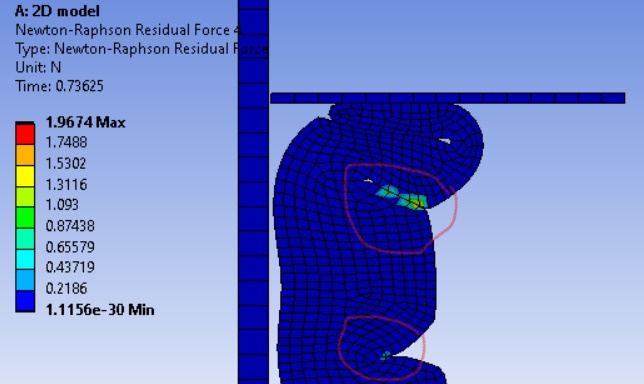I tried refining the mesh at these edges, but the solution stopped even before this point. I believe the problem at thus point is with the contact settings that I am using for self-contact, but I can figure which ones should I control or change.nnDo you have any suggestions on this?.Thank you.nnn
•peteroznewman
Subscriber
nI recommend you move this simulation to Explicit Dynamics, which has a very robust self intersection contact algorithm that is on by default in the model.nYou will need to add Density to the material.n
•Mahdi_171
Subscriber
Dear Peter, nThank you for the suggestion.Correct me if I am wrong, but isn't the excplicit solver meant to be used for impact loadings? This could mean that if I put the end time for the simulation to be like 1s (as the default in implicit), it will take hours if not days to complete the simulation. I have read before that enlarging the density will speed up the process without affecting the results to some extent for this test. Do you have any idea of this? What end time do you suggest I use for now?Thank you.
•peteroznewman
Subscriber
•peteroznewman
Subscriber
I forgot to tag you in the post above.n
•Mahdi_171
Subscriber
Dear Peter,nThank you so much. It would have taken me much more time to reach this point. I spent some time reading about the topic, and I found this equation.nThe reference says that the loading velocity should be less than 1% of the wave velocity (estimated by the previous equation) to minimize the inertia effect. I changed the elastomer to one with a higher Young's modulus to increase the wave velocity and see what happens.nI got this plot for the kinetic vs total energy.n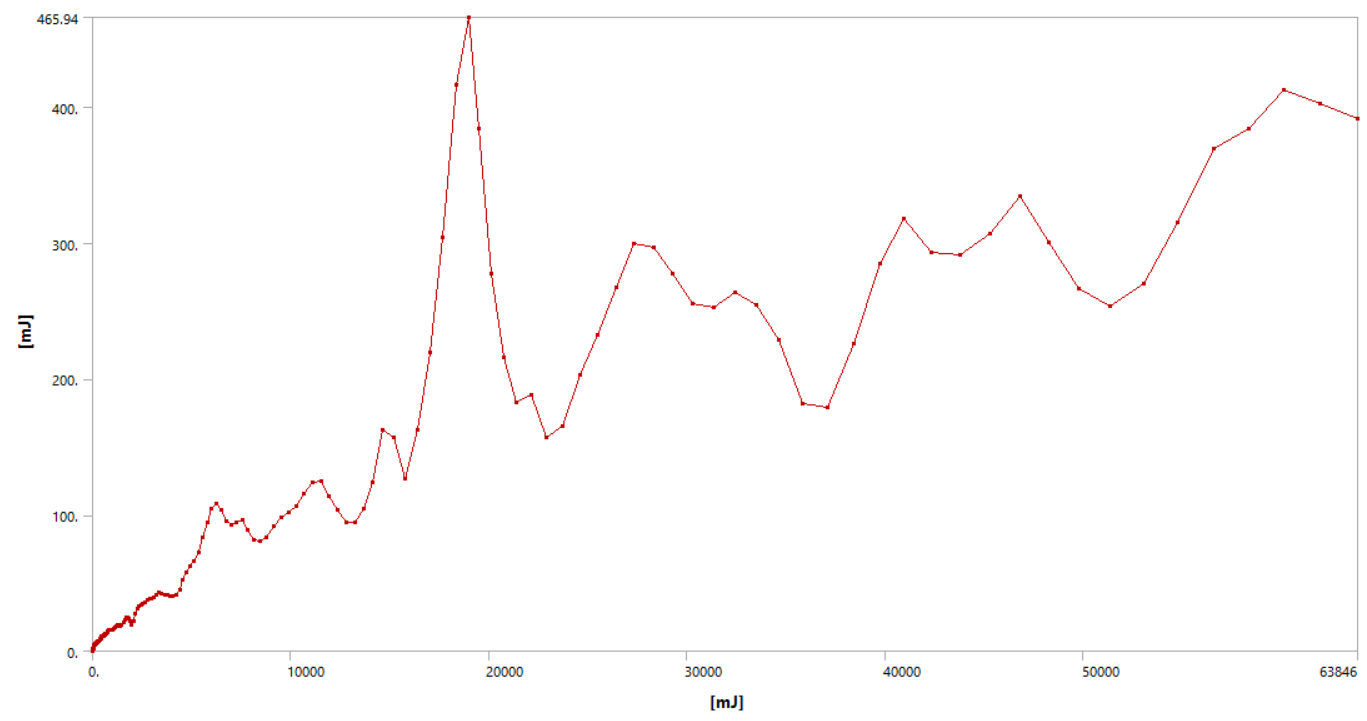I don't know if I can consider this as a quasi-static simulation, so I tried a slower one but with constant velocity, and this happened.n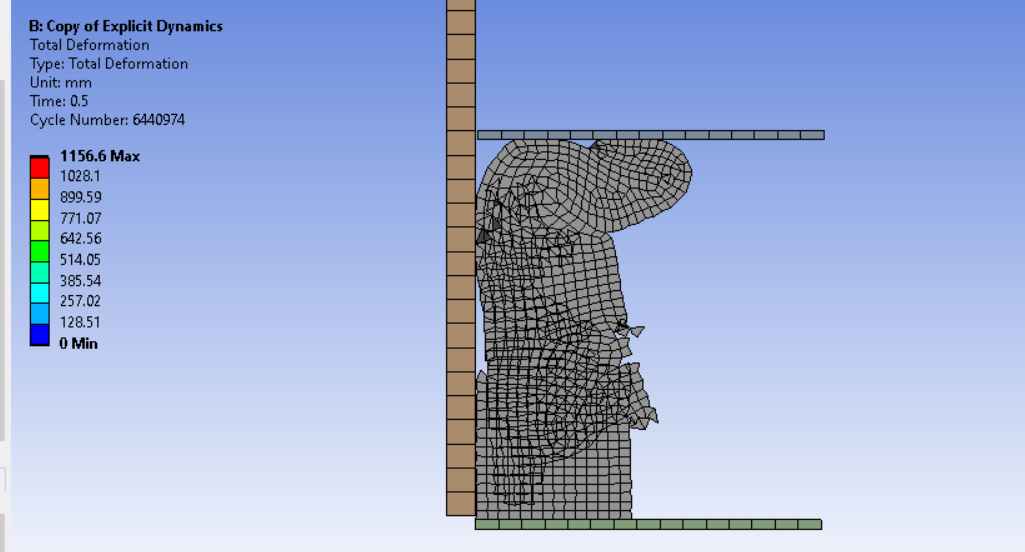nCould this be a result of high-frequency that you mentioned? The simulation was fine till a point where the elements were crushed like this, but it didn't stop and was completed to the end. The energy plot was better now, with almost zero kinetic energy before the crushing.Another thing I want to know, if possible, how can I view the deformed model in 3D. I tried to do cyclic symmetry, but the option is hidden, and the help section doesn't explain anything about that.n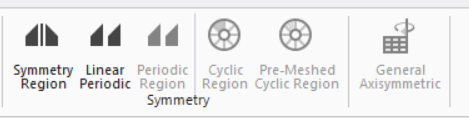•Mahdi_171
Subscriber
Dear Peter,nThank you so much. It would have taken me much more time to reach this point. I spent some time reading about the topic and I found this equation.nThe reference says that the loading velocity should be less than 1% of the material wave velocity (estimated by the previous equation) to minimize the inertia effect. I changed the elastomer to a type with higher Young's modulus to see what happens and I got this plot for the kinetic vs total energy.nI don't know if I can consider this simulation as quasi-static so I tried a slower one with constant velocity and the energy plot was much better with almost zero kinetic energy. However, the elements crushed at one point although the simulation was completed.nCould this be a result of high-frequency that you mentioned before?.I want to ask another question if possible. I want to view the 3d deformed model so I tried cyclic symmetry but the option was hidden and the help section doesn't explain anything about that.n•peteroznewman
Subscriber
nThe self contact has failed. The solution is invalid beyond that point. This is not the result of high-frequency. nCyclic symmetry is not what you use to make a 3D view of an axisymmetric model.nCyclic symmetry is to make a 3D model where the mesh repeats around an axis.n